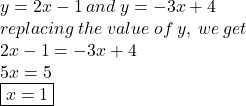## y = 2x – 1 y=-3x + 4 Solve the following system of equations for x

Question

y = 2x – 1
y=-3x + 4
Solve the following system of equations for x

in progress 0
5 months 2021-08-26T11:55:31+00:00 2 Answers 6 views 0

x = 1

General Formulas and Concepts:

Pre-Algebra

Order of Operations: BPEMDAS

1. Brackets
2. Parenthesis
3. Exponents
4. Multiplication
5. Division
7. Subtraction
• Left to Right

Equality Properties

• Multiplication Property of Equality
• Division Property of Equality
• Subtract Property of Equality

Algebra I

• Solving systems of equations using substitution/elimination

Step-by-step explanation:

Step 1: Define Systems

y = 2x – 1

y = -3x + 4

Step 2: Solve for x

Substitution

1. Substitute in y:                   2x – 1 = -3x + 4
2. Add 3x on both sides:       5x – 1 = 4
3. Add 1 to both sides:           5x = 5
4. Divide 5 on both sides:     x = 1